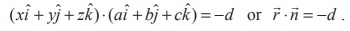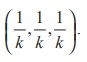Home | | Maths 12th Std | Intercept form of the equation of a plane

# Intercept form of the equation of a plane

The general equation ax + by + cz + d = 0 of first degree in x, y, z represents a plane.

Intercept form of the equation of a plane

Let the plane= q meets the coordinate axes at A,B,C respectively such that the intercepts on the axes are OA = a, OB = b, OC = c . Now position vector of the point  A is aiˆ. Since A lies on the given plane, we have  aiˆ⋅= q  which gives.Similarly, since the vectors bˆj and cˆk lie on the given plane,  we  have.  Substituting= xˆi + yˆj + zˆk in= q , we getDividing by q, we get,. This is called the intercept form of equation of the plane having intercepts a, b, c on the x, y, z axes respectively.

### Theorem 6.16

The general equation ax + by + cz + d = 0 of first degree in x, y, z represents a plane.

### Proof

The equation ax + by + cz + d = 0 can be written in the vector form as followsSince this is the vector form of the equation of a plane in standard form, the given equation ax + by + cz + d = 0 represents a plane. Here= aiˆ + bˆckˆis a vector normal to the plane.

### Note

In the general equation ax + by + cz + d = 0 of a plane, a, b, c are direction ratios of the normal to the plane.

### Example 6.38

Find the vector and Cartesian form of the equations of a plane which is at a distance of 12 units from the origin and perpendicular to 6iˆ + 2 ˆj − 3kˆ .

### Solution

Let= 6iˆ + 2 ˆj − 3kˆ and  P =12.

If dˆ is the unit normal vector in the direction of the vector 6iˆ + 2ˆj − 3kˆ , thenIfis the position vector of an arbitrary point (x, y, z) on the plane, then using.= p , the vector equation of the plane in normal form isSubstituting= xˆi + yˆj + zˆk in the above equation, we get (xˆi + yˆj + zˆk ) . 1/7 (6ˆi + 2ˆ j - 3ˆk ) = 12 .

Applying dot product in the above equation and simplifying, we get 6x + 2 y − 3z = 84, which is the the standard form.

Example 6.39

If the Cartesian equation of a plane is 3x - 4 y + 3z = -8 , find the vector equation of the plane in the standard form.

Solution

If= xi + yj + zk is the position vector of an arbitrary point (x, y, z) on the plane, then the given equation can be written as (xiˆ + yˆj + zkˆ) (3iˆ − 4 ˆj + 3kˆ) = −8 or (xiˆ + yˆj + zkˆ) (−3iˆ + 4 ˆj − 3kˆ) = 8 . That is,(−3ˆi + 4ˆj − 3ˆk ) = 8 which is the vector equation of the given plane in standard form.

Example 6.40

Find the direction cosines of the normal to the plane and length of the perpendicular from the origin to the plane(3ˆi − 4ˆ j +12ˆk ) = 5.

Solution

Let= 3iˆ − 4 ˆj +12kˆ and q = 5 .

If dˆ is the unit vector in the direction of the vector 3iˆ − 4 ˆj +12kˆ , then dˆ = 1/13 (3iˆ − 4 ˆj +12kˆ)

Now, dividing the given equation by 13 , we getwhich is the equation of the plane in the normal form.ˆd  = p

From this equation, we infer thatis a unit vector normal to the plane from the origin. Therefore, the direction cosines of dˆ  areand the length of the perpendicular from the origin to the plane is 5/13.

### Example 6.41

Find the vector and Cartesian equations of the plane passing through the point with position vector 4iˆ + 2 ˆj − 3kˆ and normal to vector 2iˆ − ˆj + kˆ .

### Solution

If the position vector of the given point is= 4i + 2 j − 3k and= 2i j + k , then the equation of the plane passing through a point and normal to a vector is given by (−)= 0 or.

Substituting= 4+ 2 − 3and= 2− k  in the above equation, we get=  (4+ 2 − 3). (2− )

Thus, the required vector equation of the plane is(2ˆi ˆj + ˆk ) = 3 . If= xˆi + yˆj + zˆk then we get the Cartesian equation of the plane 2x y + z = 3 .

### Example 6.42

A variable plane moves in such a way that the sum of the reciprocals of its intercepts on the coordinate axes is a constant. Show that the plane passes through a fixed point

### Solution

The equation of the plane having intercepts a, b, c on the x, y, z axes respectively is.

Since the sum of the reciprocals of the intercepts on the coordinate axes is a constant, we havewhere k is a constant, and which can be written asThis shows that the planepasses through the fixed pointStudy Material, Lecturing Notes, Assignment, Reference, Wiki description explanation, brief detail
12th Mathematics : UNIT 6 : Applications of Vector Algebra : Intercept form of the equation of a plane |